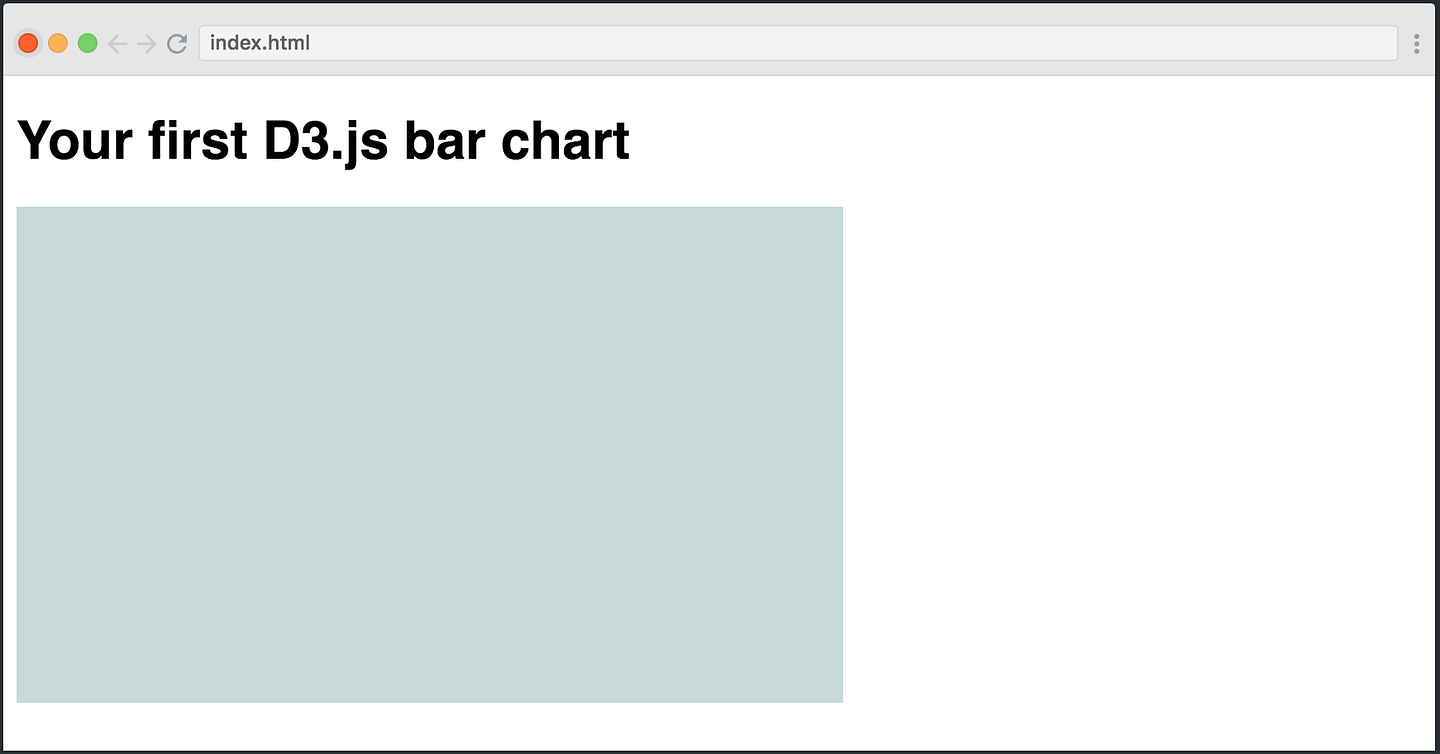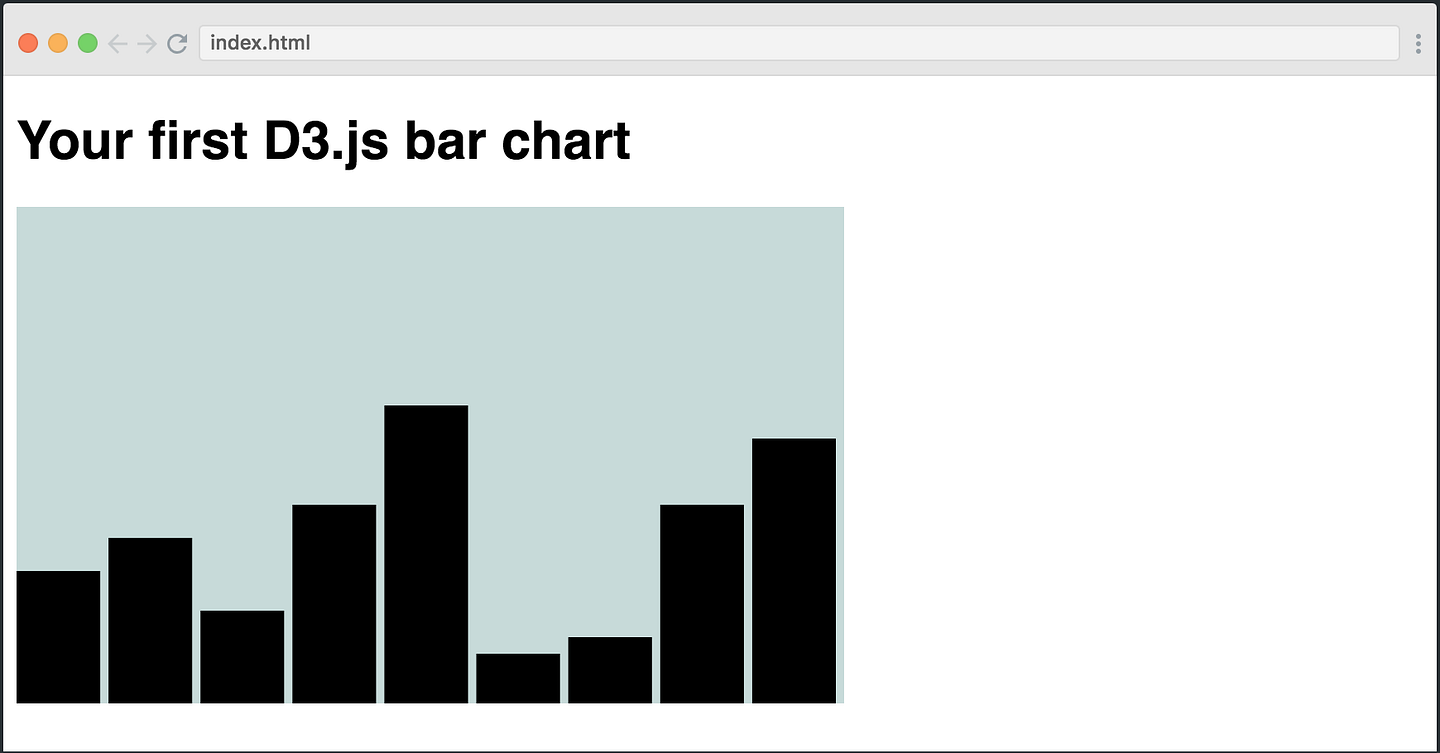# Create your first bar chart with D3.jsD3.js is the most popular JavaScript library for creating visual representations of your data. However, it’s a bit tricky to learn, so I think it’s important to start softly.

D3.js is the most popular JavaScript library for creating visual representations of your data. However, it’s a bit tricky to learn, so I think it’s important to start softly.

In this tutorial, you’ll learn how to create your very first bar chart with D3. It’ll give you an intro to the most important concepts, while still having fun building something.

We’re also creating a free D3.js course on Scrimba. Leave your email here, and we’ll give you early access once it’s launched.

Now let’s get started.

#### The setup

We’ll use the simplest setup possible, simply importing the D3 library from a CDN.

``````<html>
<body>
<svg></svg>
<script src="https://d3js.org/d3.v4.min.js"></script>
<script></script>
</body>
</html>
``````

We’ll write our D3 code in the script tag. Secondly, we’ve added an `<svg>` element to the DOM. If you want to play around with the code as you read this tutorial, check out this Scrimba playground, which contains the final version of the code.

The first thing we’re going to do is select this element, and style it a little bit.

``````var svgWidth = 500;
var svgHeight = 300;
var svg = d3.select('svg')
.attr("width", svgWidth)
.attr("height", svgHeight)
.attr("class", "bar-chart");
``````

We’re giving it a width and height, plus a `.bar-chart` class. In our CSS, we’ve styled the class like this:

``````.bar-chart {
background-color: #C7D9D9;
}
``````

Here’s the result:Now we have a nice SVG container where we can draw our bar chart. The code for doing so is a bit complex, so let’s first look at the entire thing and then walk through each step:

``````var dataset = [80, 100, 56, 120, 180, 30, 40, 120, 160];
var barWidth = (svgWidth / dataset.length);
var barChart = svg.selectAll("rect")
.data(dataset)
.enter()
.append("rect")
.attr("y", function(d) {
return svgHeight - d
})
.attr("height", function(d) {
return d;
})
.attr("transform", function (d, i) {
var translate = [barWidth * i, 0];
return "translate("+ translate +")";
});
``````

#### selectAll()

The first thing we do might seem a bit weird, we’re doing `.selectAll("rect")`, however, we haven’t yet created any `<rect>` elements yet. So this method returns an empty selection (an empty array). However, we’re going to create `<rect>` elements soon using `enter().append()`.

This might seem a bit confusing. But explaining how `selectAll()` works in combination with `enter().append()` is outside of the scope of this tutorial. If you want to understand it properly, read this article very carefully.

#### data()

We then chain the `data()` method and pass in our dataset. The data will end up dictating the height of each bar.

#### enter()

The next step is to chain the `enter()` method. The `enter()` looks both at the dataset you passed into `data()`and at the selection we did with `selectAll('rect')`, and then it tries to look for “matches.” So it creates a mapping between your data and the DOM.

But remember, the `selectAll('rect')` method returned an empty selection, as there are no `<rect>` elements in the DOM yet. However, the dataset has nine items. So there are no “matches.”

The `enter()` method then allows you to create a new `<rect>` element in the DOM for each item in the dataset which doesn’t yet have a corresponding `<rect>` element.

#### append()

On the next line, we append a `<rect>` element for each of the items. As this method follows after `enter()`, it will actually be executed nine times, one for each datapoint that lacks a corresponding `<rect>` in the DOM.

#### attr()

The next step is to decide the shape of each of the rectangles (our bars). We need to give it four attributes: height, width, x-position and y-position. We’ll use the `attr()` method for all of these.

``````.attr("y", function(d) {
return svgHeight - d
})
``````

The first parameter dictates which attribute we want to add: in this case, the y-coordinate of the bar. In the second, we get access to a callback function in which we’re going to return the value we want our attribute to have.

Here, we get access to the data point at this step of the iteration process (remember, this method is invoked once per item in the `dataset` array). The data point is stored in the `d` argument. We’ll then subtract the given datapoint, `d`, from the height of our SVG container.

X and y-coordinates are always calculated starting from the top left corner. So when we subtract the height of the container with the `d` value, we get the y-coordinate for the top of the bar.

To make the bar stretch from this point and down to the bottom of the SVG container, we’ll need to give it a height equivalent to the value of the data point:

``````.attr("height", function(d) {
return d;
})
``````

The next step is to give it a width:

``````.attr("width", barWidth - barPadding)
``````

Here, we’re simply passing in a simple expression as opposed to the callback function, as we don’t need access to the data point. We’re simply taking basis in the `barWidth` variable which we created further up, which is the total width of the container divided by the amount of bars. In order to get a small gap between each of the bars, we’ll also subtract a padding, which we’ve defined as 5.

The final step is to set the x-coordinates. This expression is a bit more complex:

``````.attr("transform", function (d, i) {
var xCoordinate = barWidth * i;
return "translate("+ xCoordinate +")";
});
``````

Here, we’re first of all taking advantage of a second parameter in the callback, `i`. This is the index of the given item in the array.

To set the coordinate for each of the bars, we’ll simply multiply the index with the `barWidth` variable. We’ll then return a string value which describes the transformation for the x-axis, for example `"translate(100)"`. That would push the bar 100 pixels to the right.

And just like that, you have your very first bar chart in D3.js.If you’re interested in learning more about D3.js, be sure to leave your email here, and we’ll send you early access to our free D3 course when it’s ready.

Recommended Courses:

Python Network Programming - Part 2: Multivendor Environment
http://bit.ly/2GLlUoR

Learn Python Prgramming Language From Beginning to Advanced
http://bit.ly/2HlJmGl

Learn to create your first website and RESTful API using Python and Flask.
http://bit.ly/2IFp89V

Python 3, be a paid scripting professional
http://on.edupioneer.net/BJGaSGm8M

Python for Data Analysis and Visualization - 32 HD Hours !
http://bit.ly/2H7G6Rn